# Colleges with the highest SAT scores in Texas

Top 10 colleges in Texas with the highest SAT scores
Looking for the colleges with the highest SAT scores in Texas? Well you're in luck! We've compiled a national college database and have created a list of the top 10 universities with the highest SAT scores in Texas. These are the schools whose applicants had the highest average SAT scores in Texas. And, since these tests are meant to determine academic prowess, they are arguably the schools with the most academically proficient students. You could even say these are the best colleges in Texas. We also include each college's ACT scores and acceptance rate so that you can see where you would have the easiest or hardest time getting in. Read on to find out more.

## Rice University SAT scores

The average SAT score for Rice University is 1505.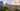The average SAT score of 1505 breaks down into:

• SAT math: 775

The average ACT score for Rice University is 34 and their acceptance rate is 11.1%.

## Southern Methodist University SAT scores

The average SAT score for Southern Methodist University is 1390.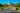The average SAT score of 1390 breaks down into:

• SAT math: 710

The average ACT score for Southern Methodist University is 31 and their acceptance rate is 47.3%.

## University of Texas at Austin SAT scores

The average SAT score for University of Texas at Austin is 1355.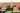The average SAT score of 1355 breaks down into:

• SAT math: 685

The average ACT score for University of Texas at Austin is 30 and their acceptance rate is 31.8%.

## Trinity University SAT scores

The average SAT score for Trinity University is 1350.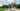The average SAT score of 1350 breaks down into:

• SAT math: 680

The average ACT score for Trinity University is 30 and their acceptance rate is 34.2%.

## University of Texas Dallas SAT scores

The average SAT score for University of Texas Dallas is 1350.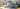The average SAT score of 1350 breaks down into:

• SAT math: 690

The average ACT score for University of Texas Dallas is 30 and their acceptance rate is 78.6%.

## Baylor University SAT scores

The average SAT score for Baylor University is 1293.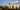The average SAT score of 1293 breaks down into:

• SAT math: 652

The average ACT score for Baylor University is 26 and their acceptance rate is 45.3%.

## Texas A&M University SAT scores

The average SAT score for Texas A&M University is 1275.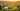The average SAT score of 1275 breaks down into:

• SAT math: 645

The average ACT score for Texas A&M University is 29 and their acceptance rate is 57.5%.

## University of Saint Thomas SAT scores

The average SAT score for University of Saint Thomas is 1265.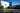The average SAT score of 1265 breaks down into:

• SAT math: 640

The average ACT score for University of Saint Thomas is 26 and their acceptance rate is 81.9%.

## Texas Christian University SAT scores

The average SAT score for Texas Christian University is 1250.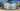The average SAT score of 1250 breaks down into:

• SAT math: 625

The average ACT score for Texas Christian University is 28 and their acceptance rate is 47.1%.

## University of Dallas SAT scores

The average SAT score for University of Dallas is 1235.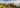The average SAT score of 1235 breaks down into:

• SAT math: 610